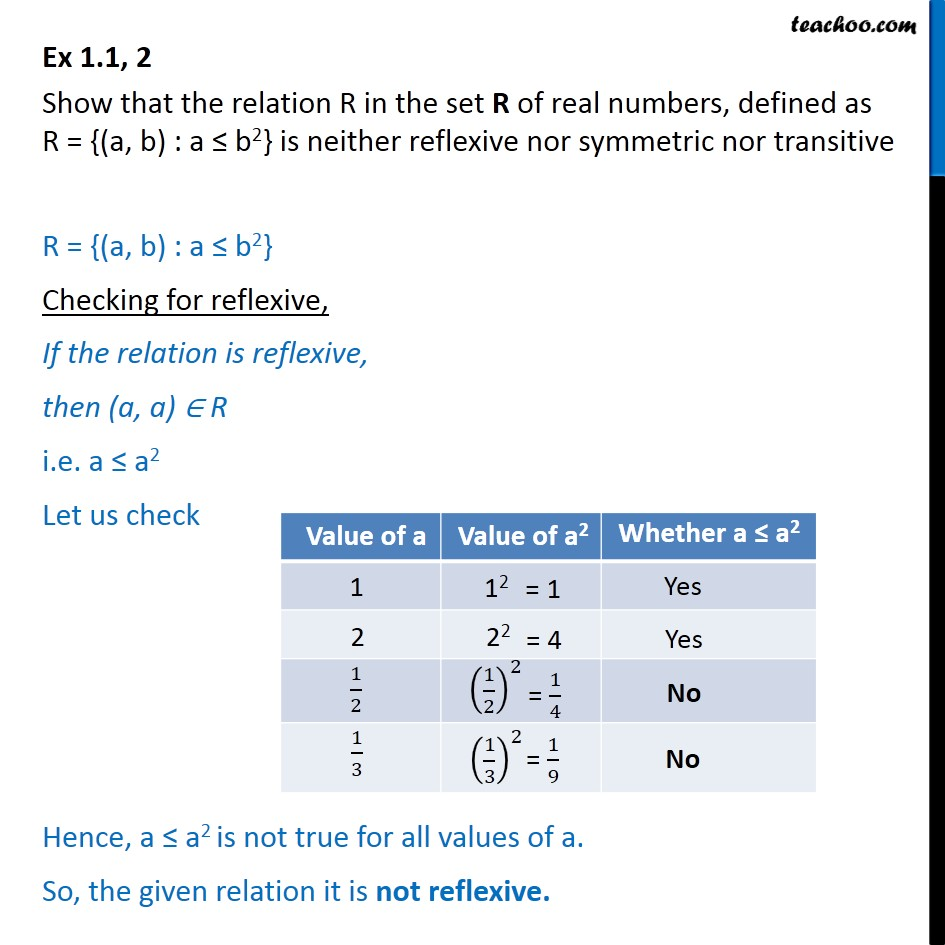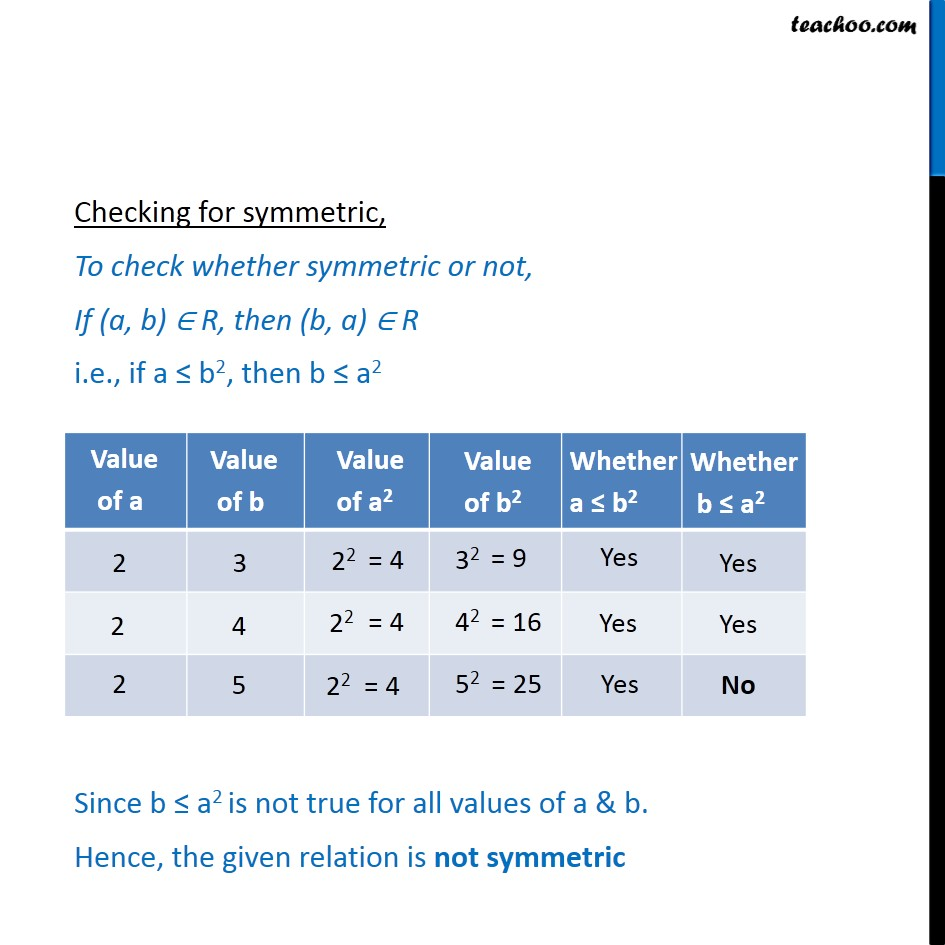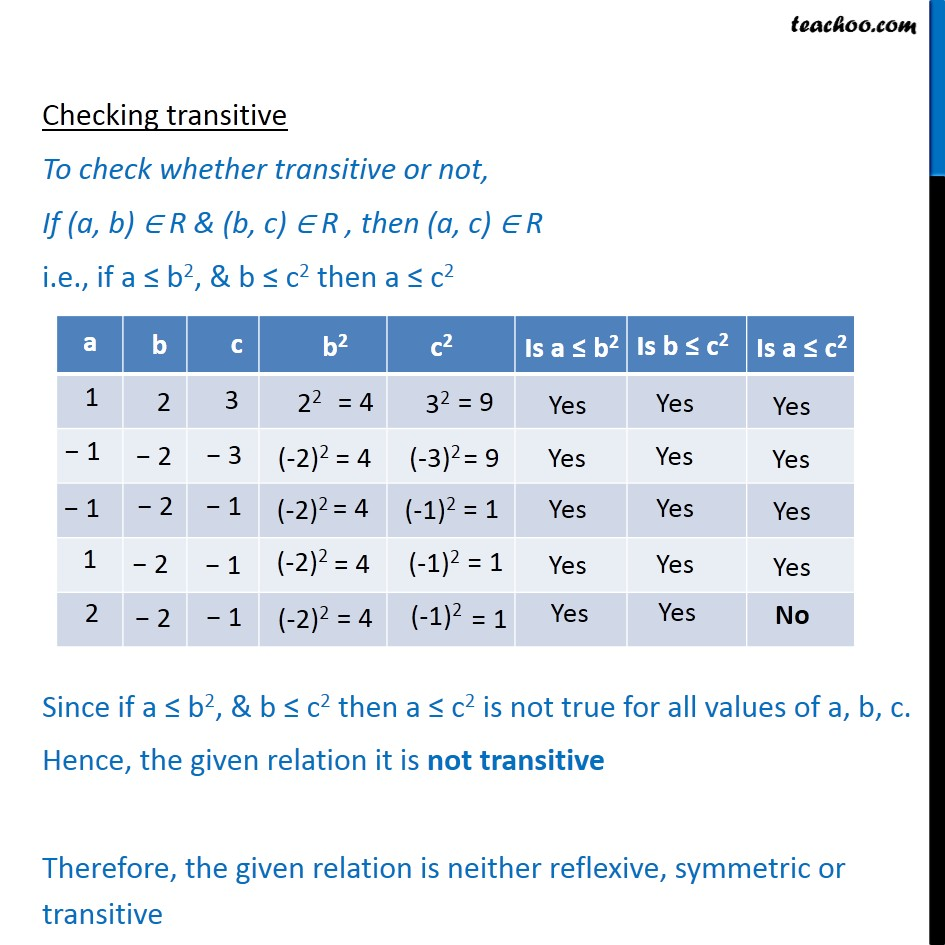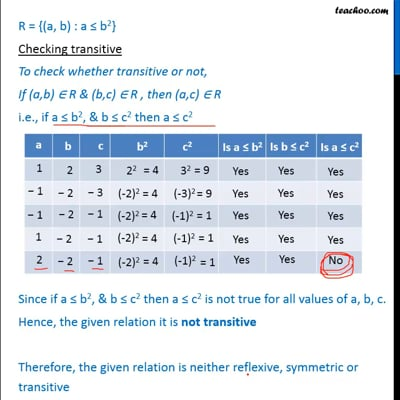To prove relation reflexive, transitive, symmetric and equivalent

Chapter 1 Class 12 Relation and Functions
Concept wiseThis video is only available for Teachoo black users

Introducing your new favourite teacher - Teachoo Black, at only ₹83 per month

### Transcript

Ex 1.1, 2 Show that the relation R in the set R of real numbers, defined as R = {(a, b) : a b2} is neither reflexive nor symmetric nor transitive R = {(a, b) : a b2} Checking for reflexive, If the relation is reflexive, then (a, a) R i.e. a a2 Let us check Hence, a a2 is not true for all values of a. So, the given relation it is not reflexive. Checking for symmetric, To check whether symmetric or not, If (a, b) R, then (b, a) R i.e., if a b2, then b a2 Since b a2 is not true for all values of a & b. Hence, the given relation is not symmetric Checking transitive To check whether transitive or not, If (a, b) R & (b, c) R , then (a, c) R i.e., if a b2, & b c2 then a c2 Since if a b2, & b c2 then a c2 is not true for all values of a, b, c. Hence, the given relation it is not transitive Therefore, the given relation is neither reflexive, symmetric or transitive